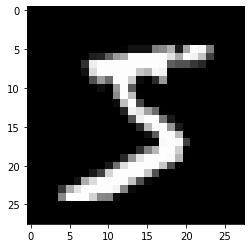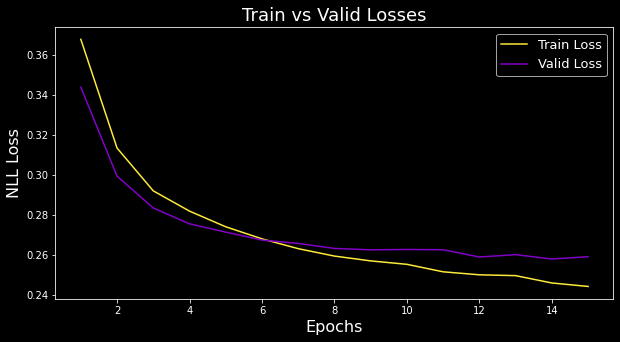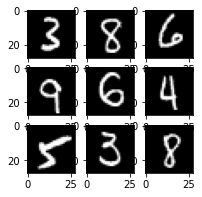## Import MNIST

Let’s download $7000$ examples of length $784 = 28\times 28$ of flattened grayscale square images of handwritten digits, stacked as a tuple of $(X,Y)$ with Python shapes $(70000, 784)$ and $(70000,).$

from sklearn.datasets import fetch_openml
mnist = fetch_openml('mnist_784', return_X_y= True)


Convert them to allowed formats and do the train-test $(5:2)$ split.

import numpy as np
X = np.float32(mnist/256) # (70000, 784)
Y = np.int64(mnist)       # (70000,)
x_train,y_train,x_valid,y_valid = X[:50000,:],Y[:50000],X[50000:,:],Y[50000:]


Take a look at the first training example!

import matplotlib.pyplot as plt
plt.imshow(x_train.reshape((28, 28)), cmap="gray")Some technical steps

1. Convert the data to “torch tensors”
2. Form a DataLoader (to simplify the iteration process over batches)

Notice that no shuffling is required for the validation data

import torch
x_train, y_train, x_valid, y_valid = map(torch.tensor,
(x_train, y_train, x_valid, y_valid))

from torch.utils.data import TensorDataset
train_ds = TensorDataset(x_train, y_train)
valid_ds = TensorDataset(x_valid, y_valid)



## Model, Optimizer & Loss

Lets design the NN architecture by subclassing  nn.Module , which manages the network parameters/weights, their gradients, etc. Create a simple linear layer  self.lin = nn.Linear(784, 10)  which implicitly is equivalent to creating a $784\times 10$ matrix of weights and a $10$-dim bias vector

self.weights = nn.Parameter(torch.randn(784, 10) / math.sqrt(784))
self.bias = nn.Parameter(torch.zeros(10))


Both parameters are initially random, Xavier initialized, but need to be determined eventually. For now, let’s not add any nonlinearities. Also incorporate the forward step, which will multiply the input by the matrix and add the bias,  xb @ self.weights + self.bias . Hence we have

from torch import nn
class FeedForward(nn.Module):
def __init__(self):
super().__init__()
self.lin = nn.Linear(784, 10)

def forward(self, xb):
return self.lin(xb)

model = FeedForward()


Pick the optimizer and the learning rate.

from torch import optim


Specify the loss.  F.cross_entropy  combines negative log likelihood loss and log softmax activation and works well for classification purposes.

import torch.nn.functional as F
loss_func = F.cross_entropy


## Training

Iterate over all training images $15$ times, each time sampling batches from DataLoader. For each batch,

1. Make a prediction model(xb), which propagates xb forward.
2. Compute the loss loss_func(pred, yb)
3. Propagate backwards:
• Optimize the weights, i.e. for each parameter p do  p -= p.grad * lr
• Reset the gradient back to $0$ so it is ready for the next batch
for epoch in range(15):
for xb, yb in train_dl:
pred = model(xb)
loss = loss_func(pred, yb) # pred.dtype=torch.float32, yb.dtype=torch.int64

loss.backward()
opt.step()
print(loss_func(model(xb), yb))


## Validation

Easy to check the validation loss too (the lines model.train() and model.eval() ensure appropriate behavior in more complex cases)

for epoch in range(15):
model.train()   # NEW
for xb, yb in train_dl:
pred = model(xb)
loss = loss_func(pred, yb)

loss.backward()
opt.step()

# Validation
model.eval()  # NEW
valid_loss = sum(loss_func(model(xb), yb).tolist() for xb, yb
in valid_dl)/ len(valid_dl)


Can also plot both losses over epochs## Make a prediction

Plot a few validation images

fig = plt.figure(figsize=(3, 3))
rows, cols = 3,3
for i in range(0, cols*rows):
plt.imshow(x_valid[i].reshape((28, 28)), cmap="gray") # True
plt.show()
print(torch.argmax(model(x_valid[0:9]), axis=1).reshape(3,3))  # Predvs $\begin{matrix} [3, 8, 6]\\ [9, 6, 4]\\ [5, 3, 8] \end{matrix}$

# CNN

Can use a Convolutional NN instead. Our inputs are $28\times 28\times 1$, where $1$ is the # of channels (only gray). The first two arguments of Conv2d are in_channels=1 and out_channels=16.

A useful formula for keeping track of dimensions is

$n_{out} = \frac{n_{in} - k + 2p}{s} + 1$

where $n$ is image’s spatial dimension (height or weight).

• Conv2d(1,16,3,2,1):    28x28x1    (28-3+2)/2+1 = 14.5    14x14x16
• Conv2d(16,16,3,2,1):   14x14x16    (14-3+2)/2+1 = 7.5    7x7x16
• Conv2d(16,10,3,2,1):   7x7x16    (7-3+2)/2+1 = 4    4x4x10
• avg_pool2d(xb,4):       4x4x10    1x10
class CNN(nn.Module):
def __init__(self):
super().__init__()
self.conv1 = nn.Conv2d(1, 16, kernel_size=3, stride=2, padding=1)
self.conv2 = nn.Conv2d(16, 16, kernel_size=3, stride=2, padding=1)
self.conv3 = nn.Conv2d(16, 10, kernel_size=3, stride=2, padding=1)

def forward(self, xb):
xb = xb.view(-1, 1, 28, 28)
xb = F.relu(self.conv1(xb))
xb = F.relu(self.conv2(xb))
xb = F.relu(self.conv3(xb))
xb = F.avg_pool2d(xb, 4)
return xb.view(-1, xb.size(1))

model = CNN()


# Full Code for CNN

# Import Data
from sklearn.datasets import fetch_openml
mnist = fetch_openml('mnist_784', return_X_y= True)

import numpy as np
X = np.float32(mnist/256) # (70000, 784)
Y = np.int64(mnist)       # (70000,)
x_train,y_train,x_valid,y_valid = X[:50000,:],Y[:50000],X[50000:,:],Y[50000:]

import matplotlib.pyplot as plt
plt.imshow(x_train.reshape((28, 28)), cmap="gray")

import torch
x_train, y_train, x_valid, y_valid = map(torch.tensor,
(x_train, y_train, x_valid, y_valid))

from torch.utils.data import TensorDataset
train_ds = TensorDataset(x_train, y_train)
valid_ds = TensorDataset(x_valid, y_valid)

# Set up architecture
from torch import nn
class CNN(nn.Module):
def __init__(self):
super().__init__()
self.conv1 = nn.Conv2d(1, 16, kernel_size=3, stride=2, padding=1)
self.conv2 = nn.Conv2d(16, 16, kernel_size=3, stride=2, padding=1)
self.conv3 = nn.Conv2d(16, 10, kernel_size=3, stride=2, padding=1)

def forward(self, xb):
xb = xb.view(-1, 1, 28, 28)
xb = F.relu(self.conv1(xb))
xb = F.relu(self.conv2(xb))
xb = F.relu(self.conv3(xb))
xb = F.avg_pool2d(xb, 4)
return xb.view(-1, xb.size(1))

model = CNN()

# Optimizer
from torch import optim

# Loss
import torch.nn.functional as F
loss_func = F.cross_entropy

# Training
for epoch in range(15):
model.train()
for xb, yb in train_dl:
pred = model(xb)
loss = loss_func(pred, yb)

loss.backward()
opt.step()

# Validation
model.eval()